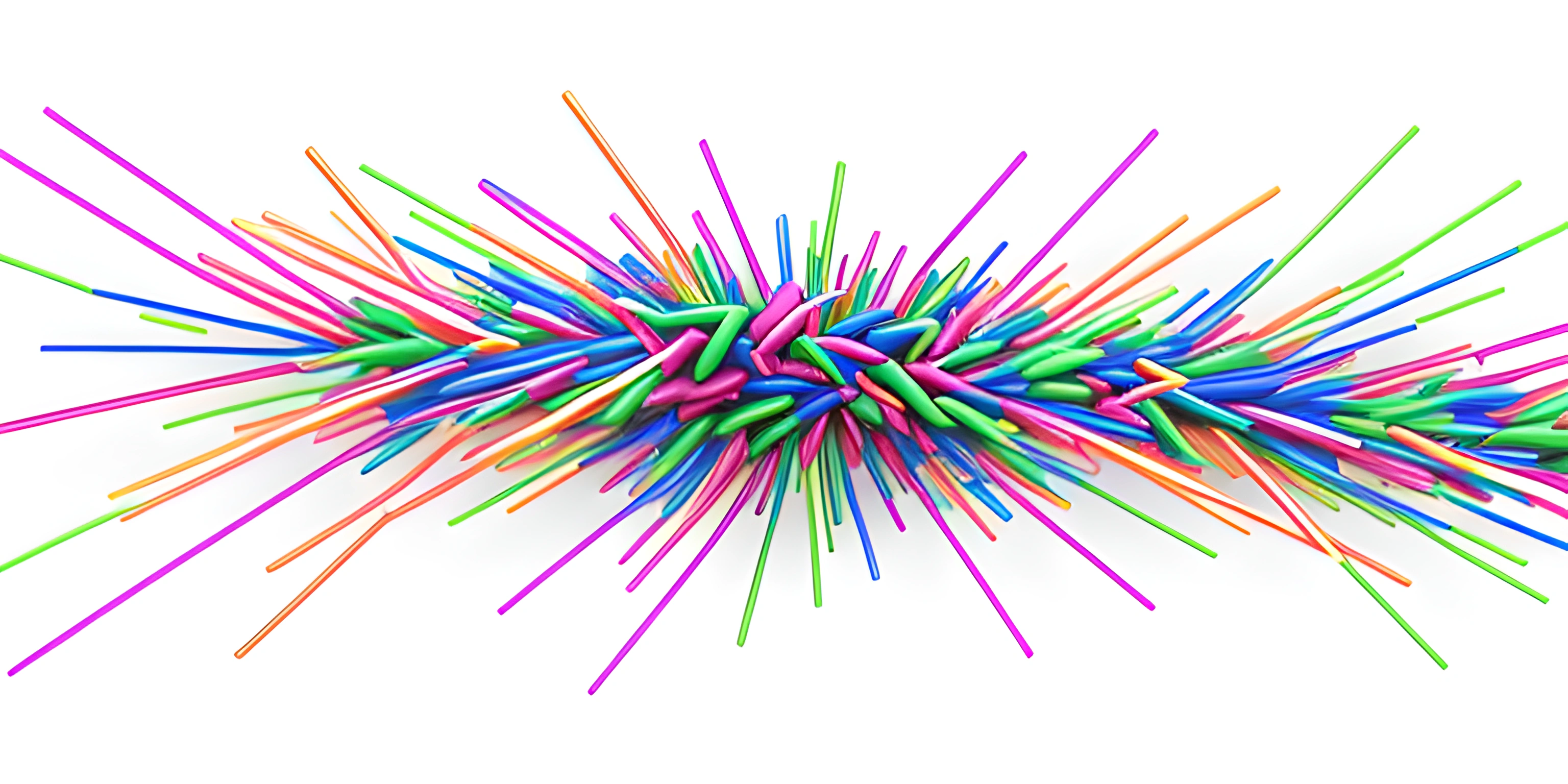# Introduction to Graphs and Their ApplicationsNote: this page has been created with the use of AI. Please take caution, and note that the content of this page does not necessarily reflect the opinion of Cratecode.

Graphs are a powerful and versatile data structure that can be used to represent complex relationships between objects. They might seem intimidating at first, but fear not! We're here to help you embark on your journey into the fascinating world of graphs, one step at a time.

## Graph Basics

A graph is a collection of vertices (also known as nodes) and edges. Vertices represent objects or entities, while edges represent relationships or connections between these objects. Think of your favorite social network, where users are the vertices, and friendships or connections between users are the edges.

### Types of Graphs

There are two main types of graphs: undirected and directed. In an undirected graph, edges have no direction, meaning connections between vertices go both ways. In a directed graph, also known as a digraph, edges have a direction, meaning connections are one-way.

For example, let's consider two friends, Alice and Bob. If we were to model their friendship using an undirected graph, we'd have an edge between Alice and Bob, representing a mutual connection. However, if we were to model their acquaintance using a directed graph, we could have an edge from Alice to Bob, indicating that Alice knows Bob, and a separate edge from Bob to Alice, indicating that Bob knows Alice.

## Graph Representation

There are multiple ways to represent graphs in programming, but the two most popular techniques are the adjacency matrix and the adjacency list.

An adjacency matrix is a 2D array (or matrix) where the cell at the ith row and jth column represents the edge between vertices i and j. If there is an edge between vertices i and j, the value of the cell is 1 (or the weight of the edge if the graph is weighted); otherwise, it's 0.

An adjacency list is an array of lists (or other data structures) where the ith element of the array contains a list of all the vertices connected to the ith vertex. This representation is more space-efficient for sparse graphs (graphs with few edges) compared to adjacency matrices.

## Applications of Graphs

Graphs are widely used in various fields and applications, including:

1. Social networks: As mentioned before, graphs are ideal for representing connections between users in social networks like Facebook, Twitter, or LinkedIn.

2. Transportation networks: Graphs can model transportation systems, such as road networks or airline routes, where cities or airports are vertices, and roads or flights are edges.

3. World Wide Web: The Internet can be seen as a giant graph, with web pages as vertices and hyperlinks as edges.

4. Project management: Directed graphs, specifically directed acyclic graphs (DAGs), can be used to model project dependencies and assist in project scheduling.

5. Biology: Graphs can represent protein-protein interactions, gene regulatory networks, or even ecological relationships between different species.

The list goes on, as graphs continue to prove themselves invaluable in unraveling complex relationships and shedding light on intricate systems.

Now that you have a basic understanding of graphs, their structure, and applications, you're well on your way to mastering this powerful data structure. Keep exploring, and don't be afraid to dive into the deep end of the graph pool!

## FAQ

### What is a graph and what are its components?

A graph is a mathematical structure used to model pairwise relations between objects. It consists of two main components: vertices (or nodes) and edges (also called arcs or lines). Vertices represent the objects, and edges represent the relations or connections between the objects.

### How are graphs classified?

Graphs can be classified based on various properties, such as:

• Directed and Undirected: In directed graphs, edges have a direction, while in undirected graphs, they do not.
• Weighted and Unweighted: In weighted graphs, edges have a numerical value (weight), while in unweighted graphs, they do not.
• Cyclic and Acyclic: Cyclic graphs contain at least one cycle (a closed path), while acyclic graphs have no cycles.
• Simple and Multigraph: Simple graphs have at most one edge between any pair of vertices, while multigraphs can have multiple edges between vertices.

### What are some common algorithms used in graph theory?

There are numerous algorithms in graph theory, some of which include:

• Depth-First Search (DFS) and Breadth-First Search (BFS) for traversing and exploring graphs
• Dijkstra's Algorithm and Bellman-Ford Algorithm for finding the shortest path between nodes in a weighted graph
• Kruskal's Algorithm and Prim's Algorithm for finding the minimum spanning tree in a connected graph
• Topological Sorting for linearly ordering vertices in a directed acyclic graph (DAG)

### How are graphs represented in computer programs?

Graphs can be represented in computer programs using two main data structures:

• Adjacency Matrix: A 2D array where the element at the i-th row and j-th column represents the edge between the i-th and j-th vertices. If an edge exists, the value will be 1 (or the edge's weight), otherwise, it will be 0.
• Adjacency List: A list of lists, where each element is a list of vertices adjacent to a given vertex. This is a more space-efficient representation, especially for sparse graphs.

### What are some real-world applications of graphs?

Graphs have numerous applications in various fields, such as:

• Computer networks, where vertices represent devices and edges represent connections
• Social networks, where vertices represent people and edges represent friendships or relationships
• Transportation systems, where vertices represent locations and edges represent routes or distances between them
• Biology, for modeling protein interactions, gene regulatory networks, and ecological relationships
• Project management, for scheduling tasks and their dependencies using a directed acyclic graph